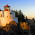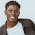## Solfa notation of 10 common Nigerian worship Songs

Do you ever dream of playing the solo of a song you are hearing for the first time, It's pretty easy, all you have to do is to train your mind and ear towards achieving it. How do you do that? By scoring out the solfa notation of almost every song you know; take these ones below as examples:

You are the pillar that Holds My life

m m m m m s f m r (2x)
m r d t l l l l l d l
t d r
m r d t l l l l l d l
t d.

Olorun to da awon oke Igbani

s d d
t d r r
d r m d t l
r r d d
t d r
l t d

m f s f m r d
t d r d t l s
m f s f m r d
d m d r

s d d
t d r r
d r m d t l
r r d d
t d r
l t d

Baba Baba Baba ooo

m s l s m r d
d m
d r d l l
d r d t t
t d r r d d

Do Oghene Doo

L....s m
d d s m
d d s r

Holy, are you Lord

d d: r m m
m f m: d r r
d d: r m m
m f m: d r r
f f m
l r d d
s m f
l l d m m r r
d f f m
l r d d
d m l
d d t d
d m l
d d t d.

Arugbo Ojo

m s s m s
m m m m f s m r
s m r d m m m m f s m r
s m r d m m m m f s m r
m r d

Ka 'bi yo Osi ooo

m r m f r d t (2x)
d d r m
r f f r d t
d r m r d l
f r d t r d

Father We declare

f f f m r
d r m r re
r m f f f r d t l t d

I will magnify the lord

d m f m f s s -call
d m f m f s s -resp
d s f m f m d -call
d s f m f m d -resp

s d l s
l l l l s
s s l l l l s
m f m r s

s d l s
l l l l s
s s l l l l s
m f m r d

Eyin ni baba; ton to Baba

d d t l l
r r d d
d d d t t
m r d

There is a material that contains over 250 Nigerian praise and worship songs and another ebook that contains the chord progression of over 150 praise and worhip songs. once you can lay your hands on playing all the solfas on your right hand and accompany them with your chords on your left hand, you would definitely be able to play praise and worship up to 75% level in the church.
To order for your own copy of the book Call or whatsapp:  09055222945
E-mail:      [email protected]

Join the Nigerian Praise and Worship instrumentalist facebook group by clicking here

Also See:

Solfa notations of 10 common Nigerian praise songs

Tonic solfa score sheet of "Bless Me" Prayer of Jabez

Tonic Solfa Score Sheet of He never failed me yet (treble, alto and tenor)

Chord Progression of 7 common Nigerian worship songs

1.Enter your comment...Wao! Thank you vErY MucH

2.This is gweat but i want more...keep it up

3.woah great job...ride on

4.5.Beautiful pieces of articles... Love it those tonic solfa

6.7.8.9.